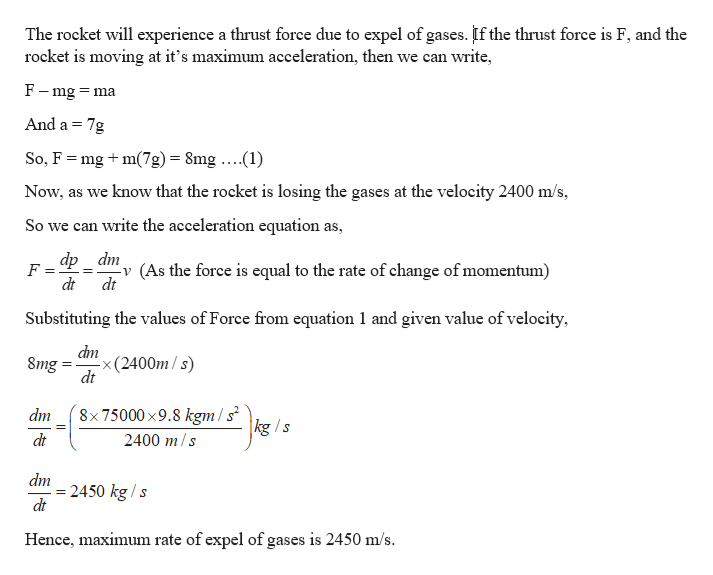# Calculate the maximum rate at which a rocket can expel gases if its acceleration cannot exceed seven times that of gravity.  The mass of the rocket just as it runs out of fuel is 75,000 kg, and its exhaust velocity is 2.40x103 m/s.  Assume that the acceleration of gravity is the same as on Earth's surface.Group of answer choices2450 kg/s2560 kg/s2670 kg/s2780 kg/s

Question
Calculate the maximum rate at which a rocket can expel gases if its acceleration cannot exceed seven times that of gravity.  The mass of the rocket just as it runs out of fuel is 75,000 kg, and its exhaust velocity is 2.40x103 m/s.  Assume that the acceleration of gravity is the same as on Earth's surface.
2450 kg/s
2560 kg/s
2670 kg/s
2780 kg/s
check_circle

Step 1

Given:

Mass of the rocket = 75000 kg

Exaust velocity of the rocket = 2400 m/s

Maximum acceleration of the rocket = 7g

Step 2

Calculating the maximum rate at which ...help_outlineImage TranscriptioncloseThe rocket will experience a thrust force due to expel of gases. If the thrust force is F, and the rocket is moving at it's maximum acceleration, then we can write F-mg = ma And a 7g So, F mgm7g)= 8mg ....(1) Now, as we know that the rocket is losing the gases at the velocity 2400 m/s So we can write the acceleration equation as, dp v (As the force is equal to the rate of change of momentum) dt dm dt Substituting the values of Force from equation 1 and given value of velocity, dm -x(2400m/s) dt 8mg= 8x 75000 x9.8 kgm/s |kg /s dm 2400 m/s dt dm = 2450 kg /s dt Hence, maximum rate of expel of gases is 2450 m/s. fullscreen

### Want to see the full answer?

See Solution

#### Want to see this answer and more?

Solutions are written by subject experts who are available 24/7. Questions are typically answered within 1 hour.*

See Solution
*Response times may vary by subject and question.
Tagged in

### Physics